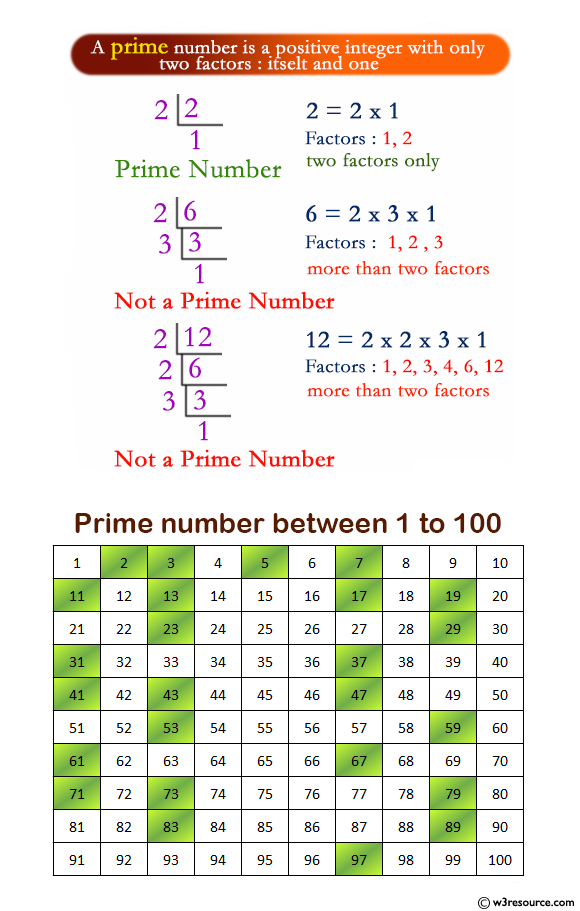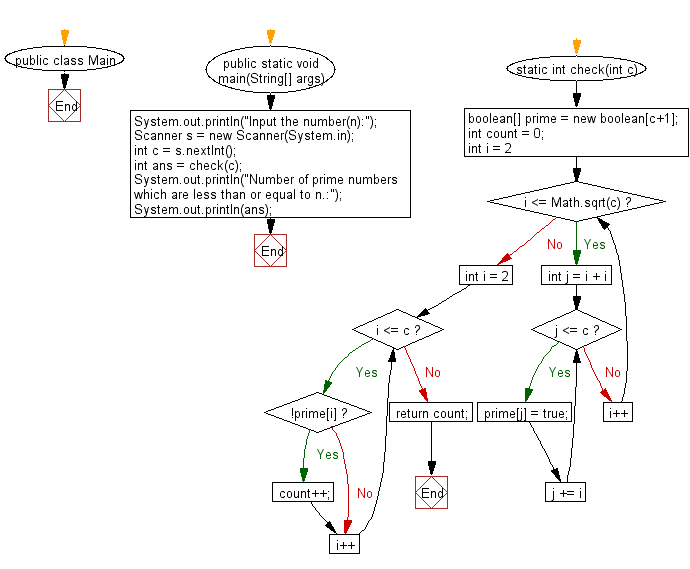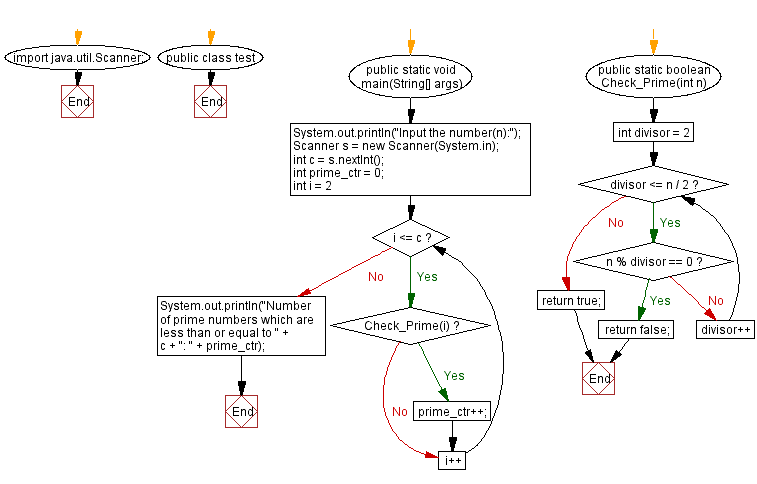# Java: Print the number of prime numbers which are less than or equal to a given integer

## Java Basic: Exercise-217 with Solution

Write a Java program to print the number of prime numbers less than or equal to a given integer.

Input:

n (1 ≤ n ≤ 999,999).

Pictorial Presentation:Sample Solution-1:

Java Code:

`````` import java.util.Scanner;
public class Main {

public static void main(String[] args) {
System.out.println("Input the number(n):");
Scanner s = new Scanner(System.in);
int c = s.nextInt();
int ans = check(c);
System.out.println("Number of prime numbers which are less than or equal to n.:");
System.out.println(ans);
}
static int check(int c) {
boolean[] prime = new boolean[c+1];
int count = 0;
for(int i = 2; i <= Math.sqrt(c); i++) {
for(int j = i + i; j <= c; j += i) {
prime[j] = true;
}
}
for(int i = 2; i <= c; i++) {
if(!prime[i]) {
count++;
}
}
return count;
}
}

```
```

Sample Output:

```Input the number(n):
1235
Number of prime numbers which are less than or equal to n.:
202
```

Flowchart:Sample Solution-2:

Java Code:

``````import java.util.Scanner;
public class test {
public static void main(String[] args) {
System.out.println("Input the number(n):");
Scanner s = new Scanner(System.in);
int c = s.nextInt();
int prime_ctr = 0;
for (int i = 2; i <= c; i++) {
if (Check_Prime(i)) {
prime_ctr++;
}
}
System.out.println("Number of prime numbers which are less than or equal to " + c + ": " + prime_ctr);
}
public static boolean Check_Prime(int n) {
for (int divisor = 2; divisor <= n / 2; divisor++) {
if (n % divisor == 0) {
return false;
}
}
return true;
}
}
```
```

Sample Output:

```Input the number(n):
1235
Number of prime numbers which are less than or equal to 1235: 202
```

Flowchart:Java Code Editor: